# Oberseminar Analysis Summer 2017

## Organizers: A. Schlichting, S. Conti, H. Koch, S. Müller, B. Niethammer, M. Rumpf, C. Thiele, J.J.L. Velázquez

• Thursday, March 23, 2:15 p.m., Lipschitz-Saal
Fulvio Ricci (Scuola Normale Superiore, Pisa)
A maximal restriction theorem and Lebesgue points of Fourier transforms in the plane
We present the results of recent joint work with Detlef Müller and James Wright. A Fourier restriction theorem relative to a given surface $S\subset \mathbb R^n$ provides $L^p-L^q$ inequalities for the operator $\mathcal R:f\in\mathcal S(\mathbb R^n)\longmapsto \hat f_{|_S}$. Despite the wide literature concerning range of validity and applications of restriction inequalities, not much has been said about the explicit relation, in presence of a $p-q$ restriction inequality, between the two functions $\hat f$ and $\mathcal R f$ for a general $f\in L^p(\mathbb R^n)$. We give a first partial answer for curves in the plane by analyzing the operator which assigns to $f\in L^p(\mathbb R^2)$, with $p<4/3$, a smoothened version of the strong maximal function of $\hat f$.

• Thursday, April 20, 2:15 p.m., Lipschitz-Saal
Francesco Fanelli (Institut Camille Jordan, Université de Lyon)
Asymptotic behaviour of non-homogenous fluids in fast rotation
In this talk, we consider a class of singular perturbation problems for systems of PDEs related to the dynamics of geophysical fluids. We are interested here in effects due to both the non-homogeneity of the fluid and the Earth rotaton, and to their interplay. After a review of known results, we specilize on the 2-D density-dependent incompressible Navier-Stokes equations with Coriolis force: our goal is to characterize the asymptotic dynamics of weak solutions to this model, in the limit when the rotation becomes faster and faster.
We present two kinds of results (deeply different from each other, from a qualitative viewpoint), depending on whether the initial densities are small perturbations of a constant state or of a truly non-constant reference density. In the former case we prove that the system tends to a homogeneous Navier-Stokes system with an additional forcing term, which is due to density variations and which is a remainder of the action of the Coriolis force. In the latter case, instead, we show that the limit equations become linear, and moreover one can identify only a mean motion, in terms of the limit vorticity and the limit density fluctuation function; this issue can be interpreted as a sort of turbulent behaviour of the fluid in the limit of fast rotation.
This talk is based on a joint work with Isabelle Gallagher.

• Thursday, April 27, 2:15 p.m., seminar room 0.008
Diogo Oliveira e Silva (University of Bonn)
Some recent progress on sharp Fourier restriction theory
It has long been understood that Strichartz estimates for the homogeneous Schrödinger equation correspond to adjoint restriction estimates on the paraboloid. The study of extremizers and sharp constants for the corresponding inequalities has a short but rich history. In this talk, I will summarize it briefly, and then specialize to the case of certain convex perturbations of the paraboloid. A geometric comparison principle for convolution measures can be used to establish the corresponding sharp Strichartz inequality, and to prove that extremizers do not exist. The mechanism underlying this lack of compactness is explained by the behaviour of extremizing sequences which will be described via concentration-compactness. Time permitting, I will show how this resolves a dichotomy from the recent literature concerning the existence of extremizers for a family of fourth order Schrödinger equations.

• Thursday, May 4, 2:15 p.m., Lipschitz-Saal
Christian Seis (University of Bonn)
Optimal stability estimates for continuity equations
In this talk, I will review new stability estimates for continuity equations and compare those with previosuly known analogous estimates for Lagrangian flows. I will explain how the new results allow for a quantitive proof of well-posedness in the low regularity setting considered by DiPerna and Lions. The estimates are obtained for Kantorovich--Rubinstein distances with logarithmic cost functions and allow thus to quantify the order of weak convergence in several applications. I plan to conclude this talk with two examples: 1) A lower bound on mixing rates obtained by stirring two immiscible fluids. 2) An upper bound on convergence rates for numerical upwind schemes (obtained jointly with A. Schlichting).

• Thurday, June 1, 2:15 p.m., seminar room 0.008
Charlotte Perrin (RWTH Aachen)
A macroscopic model for granular flows
I will present in this talk an original model for immersed granular flows which takes into account memory effects. In the first part I will justify these memory effects by means of a singular limit. It relies on recent analysis tools for the compressible Navier-Stokes equations. The second part of the talk will be dedicated to one-dimensional flows for which a direct Lagrangian approach can be developed.

• June 20 - 29, 2017
Robert L. Pego (Carnegie Mellon University)
Lipschitz Lectures: Studies in dynamics, coherent structures and stability

• Thursday, July 6, 2:15 p.m., seminar room 0.008
Simon Rösel (WIAS Berlin)
Density of convex intersections and applications
In a general framework, it is shown how density properties of intersections of convex sets naturally arise from the perturbation or dualization of constrained optimization and variational inequality problems. Several density results (and counterexamples) for closed convex sets with pointwise constraints in Sobolev spaces are presented. Diverse applications are provided, which include elasto-plasticity and image restoration problems. Finally, the results are further discussed in the context of Finite Element discretizations of sets associated to convex constraints.

• Thursday, July 13, 2:15 p.m., Lipschitz-Saal
Ievgen Verbytskyi (National University of Ukraine)
A model of tissue border displacement in non-contact Photoacoustic Tomography
Photoacoustic tomography (PAT) is a relatively new imaging modality, which allows e.g. to visualize the vascular network in biological tissue noninvasively. This tomographic method has an advantage in comparison to pure optical/acoustical methods due to high optical contrast and low acoustic scattering in deep tissue. The common PAT methodology, based on measurements of the acoustic pressure by piezoelectric sensors placed on the tissue surface, limits its practical versatility. A novel, completely non-contact and full-field PAT system is described. In noncontact PAT the measurement of surface displacement induced by the acoustic pressure at the tissue/air border is researched. A model of the tissue displacement caused by medium pressure based on the momentum conservation law is proposed. Experimental data processing and simulation techniques are developed. The error of the displacement simulation in comparing with experimental data is calculated.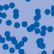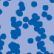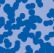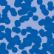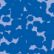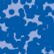## News

Florian Schweiger erhielt den Hausdorff-Gedächtnispreis 2021 der Fachgruppe Mathematik für die beste Dissertation. Er fertigte die Dissertation unter der Betreuung von Prof. Stefan Müller an. Unter anderen wurde Vanessa Ryborz mit einem Preis der Bonner Mathematischen Gesellschaft für ihre von Prof. Sergio Conti betreute Bachelorarbeit ausgezeichnet. (18.01.2022)

Prof. Dr. Sergio Albeverio has been elected into the Academia Europaea and the Accademia Nazionale dei Lincei (more; 02.12.2021).

Der SFB 1060 Die Mathematik der emergenten Effekte hat eine dritte Förderperiode erhalten. (26.11.20)

Prof. Dr. Andreas Eberle erhält den diesjährigen Lehrpreis der Universität Bonn. (22.07.2020)

Herr Dr. Richard Höfer erhielt den Hausdorff-Gedächtnispreis 2019 der Fachgruppe Mathematik für die beste Disseration. Betreut wurde die Arbeit von Prof. J. Velázquez (29.01.2020).

Contact

Managing Director: Prof. Dr. Massimiliano Gubinelli